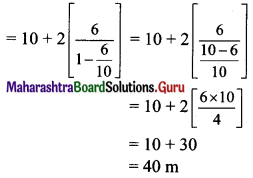Balbharti Maharashtra State Board 11th Maths Book Solutions Pdf Chapter 2 Sequences and Series Ex 2.3 Questions and Answers.

## Maharashtra State Board 11th Maths Solutions Chapter 2 Sequences and Series Ex 2.3

Question 1.
Determine whether the sum to infinity of the following G.P.s exist, if exists find them.
(i) $$\frac{1}{2}, \frac{1}{4}, \frac{1}{8}, \frac{1}{16}, \ldots$$
Solution: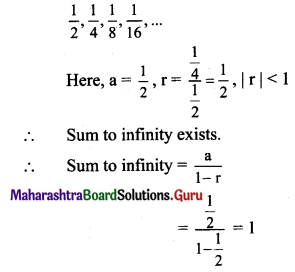(ii) $$2, \frac{4}{3}, \frac{8}{9}, \frac{16}{27}, \ldots$$
Solution: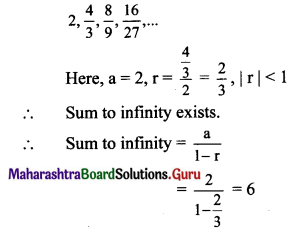(iii) $$-3,1, \frac{-1}{3}, \frac{1}{9}, \ldots$$
Solution: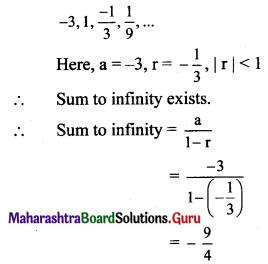(iv) $$\frac{1}{5}, \frac{-2}{5}, \frac{4}{5}, \frac{-8}{5}, \frac{16}{5}, \ldots$$
Solution: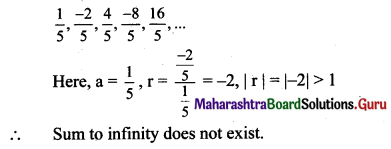(v) 9, 8.1, 7.29, ……
Solution:
9, 8.1, 7.29, …..
Here, a = 9, r = $$\frac{8.1}{9}$$ = 0.9, |r| < 1
∴ Sum to infinity exists.
∴ Sum to infinity = $$\frac{\mathrm{a}}{1-\mathrm{r}}$$
= $$\frac{9}{1-0.9}$$
= $$\frac{9}{0.1}$$
= 90

Question 2.
Express the following recurring decimals as rational numbers.
(i) $$0 . \overline{7}$$
(ii) $$2 . \overline{4}$$
(iii) $$2.3 \overline{5}$$
(iv) $$51.0 \overline{2}$$
Solution:
(i) $$0 . \overline{7}$$ = 0.7777… = 0.7 + 0.07 + 0.007 + ….
The terms 0.7, 0.07, 0.007,… are in G.P.
∴ a = 0.7, r = $$\frac{0.07}{0.7}$$ = 0.1, |r| = |0.1| < 1
∴ Sum to infinity exists.
∴ Sum to infinity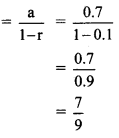(ii) $$2 . \overline{4}$$ = 2.444 … = 2 + 0.4 + 0.04 + 0.004 + …
The terms 0.4, 0.04, 0.004,… are in G.P.
∴ a = 0.4, r = $$\frac{0.07}{0.7}$$ = 0.1, |r| = 10.11 < 1
∴ Sum to infinity exists.
∴ Sum to infinity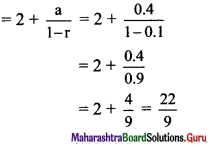(iii) $$2.3 \overline{5}$$ = 2.3555… = 2.3 + 0.05 + 0.005 + 0.0005 + …
The terms 0.05,0.005,0.0005,… are in G.P.
∴ a = 0.05, r = $$\frac{0.005}{0.05}$$ = 0.1, |r| = |0.1| < 1
∴ Sum to infinity exists.
∴ Sum to infinity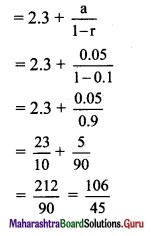(iv) $$51.0 \overline{2}$$ = 51.0222 …. = 51 + 0.02 + 0.002 + 0.0002 + …..
The terms 0.02, 0.002, 0.0002,… are in G.P.
∴ a = 0.02, r = $$\frac{0.002}{0.02}$$ = 0.1, |r| = |0.1| < 1
∴ Sum to infinity exists.
∴ Sum to infinity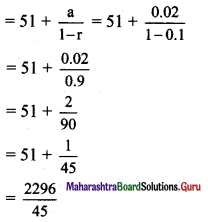Question 3.
If the common ratio of a G.P. is $$\frac{2}{3}$$ and the sum to infinity is 12, find the first term.
Solution:
r = $$\frac{2}{3}$$, sum to infinity = 12 ….. [Given]
Sum to infinity = $$\frac{\mathrm{a}}{1-\mathrm{r}}$$
12 = $$\frac{a}{1-\frac{2}{3}}$$
a = 12 × $$\frac{1}{3}$$
∴ a = 4

Question 4.
If the first term of the G.P. is 6 and its sum to infinity is $$\frac{96}{17}$$, find the common ratio.
Solution:
a = 6, sum to infinity = $$\frac{96}{17}$$ …..[Given]
Sum to infinity = $$\frac{\mathrm{a}}{1-\mathrm{r}}$$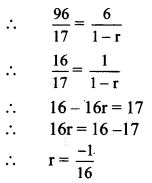Question 5.
The sum of an infinite G.P. is 5 and the sum of the squares of these terms is 15, find the G.P.
Solution:
Let the required G.P. be a, ar, ar2, ar3, …..
Sum to infinity of this G.P. = 5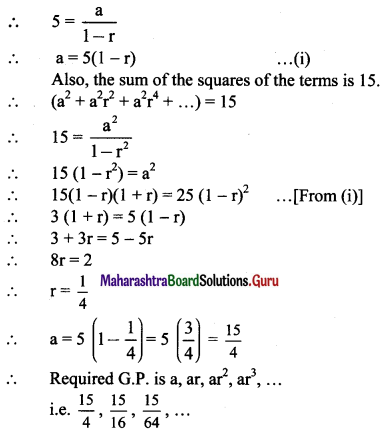Question 6.
Find
(i) $$\sum_{r=1}^{\infty} 4(0.5)^{r}$$
Solution: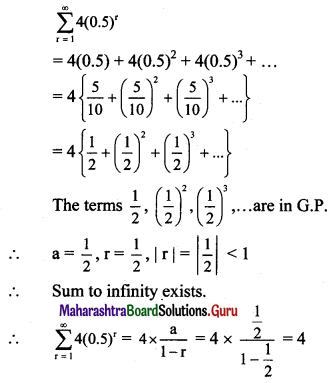(ii) $$\sum_{r=1}^{\infty}\left(-\frac{1}{3}\right)^{r}$$
Solution: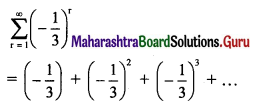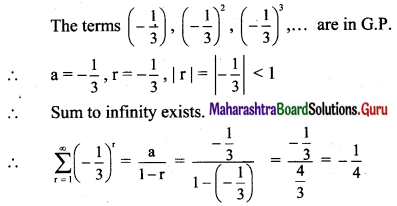(iii) $$\sum_{r=0}^{\infty}(-8)\left(-\frac{1}{2}\right)^{r}$$
Solution: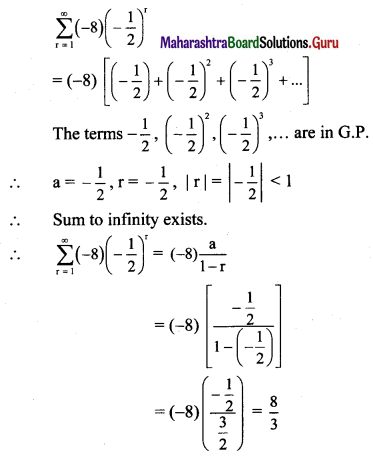(iv) $$\sum_{n=1}^{\infty} 0.4^{n}$$
Solution: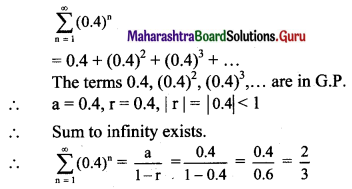Question 7.
The midpoints of the sides of a square of side 1 are joined to form a new square. This procedure is repeated indefinitely. Find the sum of
(i) the areas of all the squares.
(ii) the perimeters of all the squares.
Solution: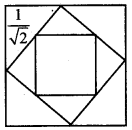(i) Area of the 1st square = 12
Area of the 2nd square = $$\left(\frac{1}{\sqrt{2}}\right)^{2}$$
Area of the 3rd square = $$\left(\frac{1}{2}\right)^{2}$$
and so on.
∴ Sum of the areas of all the squares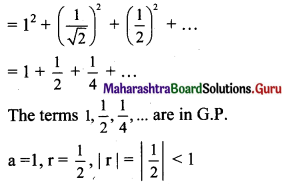∴ Sum to infinity exists.
∴ Sum of the areas of all the squares = $$\frac{1}{1-\frac{1}{2}}$$ = 2

(ii) Perimeter of 1st square = 4
Perimeter of 2nd square = 4$$\left(\frac{1}{\sqrt{2}}\right)$$
Perimeter of 3rd square = 4$$\left(\frac{1}{2}\right)$$
and so on.
Sum of the perimeters of all the squares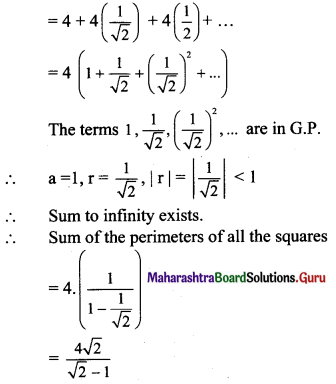Question 8.
A ball is dropped from a height of 10 m. It bounces to a height of 6m, then 3.6 m, and so on. Find the total distance travelled by the ball.
Solution:
Here, on the first bounce, the ball will go 6 m and it will return 6 m.
On the second bounce, the ball will go 3.6 m and it will return 3.6 m, and so on.
Given that, a ball is dropped from a height of 10 m.
∴ Total distance travelled by the ball is = 10 + 2[6 + 3.6 + …]
The terms 6, 3.6 … are in G.P.
a = 6, r = 0.6, |r| = |0.6| < 1
∴ Sum to infinity exists.
∴ Total distance travelled by the ball